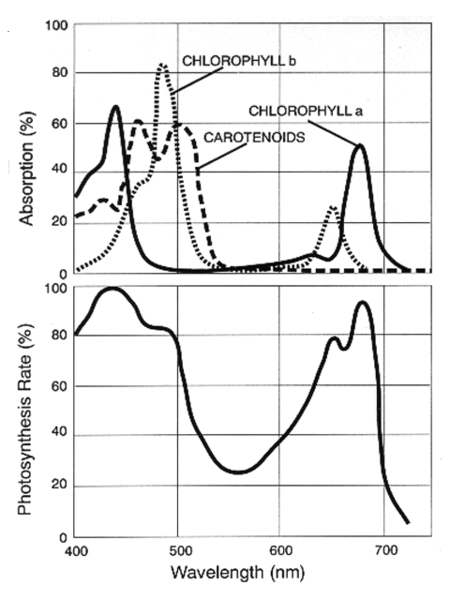# 5.4.1: Biology- The Wave Model for Light and Electrons

$$\newcommand{\vecs}{\overset { \rightharpoonup} {\mathbf{#1}} }$$ $$\newcommand{\vecd}{\overset{-\!-\!\rightharpoonup}{\vphantom{a}\smash {#1}}}$$$$\newcommand{\id}{\mathrm{id}}$$ $$\newcommand{\Span}{\mathrm{span}}$$ $$\newcommand{\kernel}{\mathrm{null}\,}$$ $$\newcommand{\range}{\mathrm{range}\,}$$ $$\newcommand{\RealPart}{\mathrm{Re}}$$ $$\newcommand{\ImaginaryPart}{\mathrm{Im}}$$ $$\newcommand{\Argument}{\mathrm{Arg}}$$ $$\newcommand{\norm}{\| #1 \|}$$ $$\newcommand{\inner}{\langle #1, #2 \rangle}$$ $$\newcommand{\Span}{\mathrm{span}}$$ $$\newcommand{\id}{\mathrm{id}}$$ $$\newcommand{\Span}{\mathrm{span}}$$ $$\newcommand{\kernel}{\mathrm{null}\,}$$ $$\newcommand{\range}{\mathrm{range}\,}$$ $$\newcommand{\RealPart}{\mathrm{Re}}$$ $$\newcommand{\ImaginaryPart}{\mathrm{Im}}$$ $$\newcommand{\Argument}{\mathrm{Arg}}$$ $$\newcommand{\norm}{\| #1 \|}$$ $$\newcommand{\inner}{\langle #1, #2 \rangle}$$ $$\newcommand{\Span}{\mathrm{span}}$$$$\newcommand{\AA}{\unicode[.8,0]{x212B}}$$

We noted that light, color, and photochemistry owe their existence to the electronic structure of atoms. It may not be surprising, therefore, that the same wave/particle model that is used for light can be applied to electronic structure, and that we can get some insight into the energy involved in photosynthesis by studying the light that is absorbed. Let's see how this wave model developed, and review the wave model.

### Energy for Photosynthesis

Not all light is effective in allowing plants to carry out photosynthesis. Plants generally absorb light in roughly the same region that is visible to the Human eye (350 - 700 nm). Shorter wavelengths are absorbed by the ozone layer, or if they make it through, have enough energy to cause cell damage. Longer wavelengths do not provide enough energy for photosynthesis.

##### Example $$\PageIndex{2}$$: Energy of Photons

The absorbance spectrum of some biomolecules involved in photosynthesis is shown here.Two graphs are presented. One is the photosynthesis rate in percentage against wavelength in nanometers. This graph has only one curve with multiples ups and downs. Absorption rate in percentage is also shown against wavelength in nanometers. Absorption spectrum for chlorophyll b, chlorophyll a, and carotenoids are shown. Each curve also has multiple up and downs.
Figure $$\PageIndex{7}$$ Photosynthetic Action Spectrum 

Note that "chlorophyll a" absorbs strongly in the red at ~680 nm and in the blue at ~440 nm. Photosynthesis cannot occur in plants irradiated with light in the yellow region (570-590 nm), because it is not absorbed, no matter how bright it is. What is the energy of photons with a wavelength of 440 nm?

Solution:

$$\text{E} = \text {h} \times \nu =\frac{\text{h}\times\text{c}}{\lambda}$$

$$\text{E} = [(6.626 \times 10^{-34} \text{ J s} )(3 \times 10^{8})] / 440 \times 10^{-9} \times m = 4.52 \times 10^{-19} \text{J}$$

The energy supplied by each photon of 680 nm light is 2.92 x 10-19 J, and that of 580 nm light is 3.43 x 10-19 J. Even though it lies between the energies of the two photons that cause photosynthesis, it is completely ineffective, no matter how bright (how many photons per second).

In the early 1900s Frederick Frost Blackman and Gabrielle Matthaei<rf>en.Wikipedia.org/wiki/Photosynthesis</ref> found that at constant temperature, the rate of carbon assimilation varies with light intensity, initially increasing as the intensity increases. How can this be consisten with the energy of light being related to just its frequency or wavelength?

The paradox is solved by noting that intensity is the number of photons per second. If one photon has enough energy to initiate photosynthesis in one chlorophyll molecule, then many photons will cause more photosynthesis. But if the photons were 580 nm light, they would have no effect, no matter what their intensity.

Some botanists claim that blue light at 440 nm is most effective in promoting leaf growth through photosynthesis, while red light around 680 nm is most effective in causing flowering.

## References

1. ↑ en.Wikipedia.org/wiki/Wavelenght
2. ↑ en.Wikipedia.org/wiki/Photosynthesis